# 1. 前言

``````  let array = [1,2,3];
array = [];
``````

``````    let array = reactive([1,2,3]);
watch(()=>[...array],()=>{
console.log(array);
},)
array = reactive([]);
``````

# 2. 清空数据的几种方式

## 2.1 使用ref()

``````    const array = ref([1,2,3]);

watch(array,()=>{
console.log(array.value);
},)

array.value = [];
``````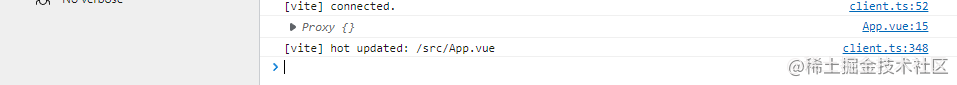## 2.2 使用slice

`slice`顾名思义，就是对数组进行`切片`，然后返回一个新`数组`,感觉和`go`语言的`切片`有点类似。当然用过`react`的小伙伴应该经常用`slice`，清空一个数组只需要这样写:

``````    const array = ref([1,2,3]);

watch(array,()=>{
console.log(array.value);
},)

array.value = array.value.slice(0,0);
``````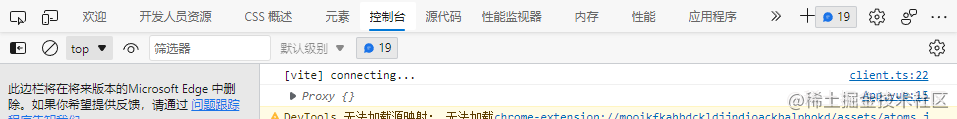不过需要注意要使用`ref`

## 2.3 length赋值为0

``````    const array = ref([1,2,3]);

watch(array,()=>{
console.log(array.value);
},{
deep:true
})

array.value.length = 0;
``````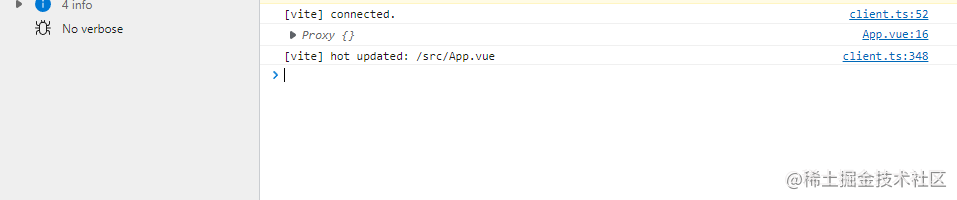``````    const array = reactive([1,2,3]);

watch(()=>[...array],()=>{
console.log(array);
})

array.length = 0;
``````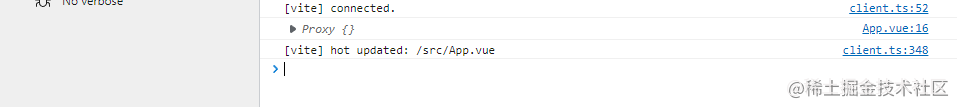## 2.4 使用splice

`副作用`函数`splice`也是一种方案，这种情况同时也可以使用`reactive`:

``````    const array = reactive([1,2,3]);

watch(()=>[...array],()=>{
console.log(array);
},)

array.splice(0,array.length)
``````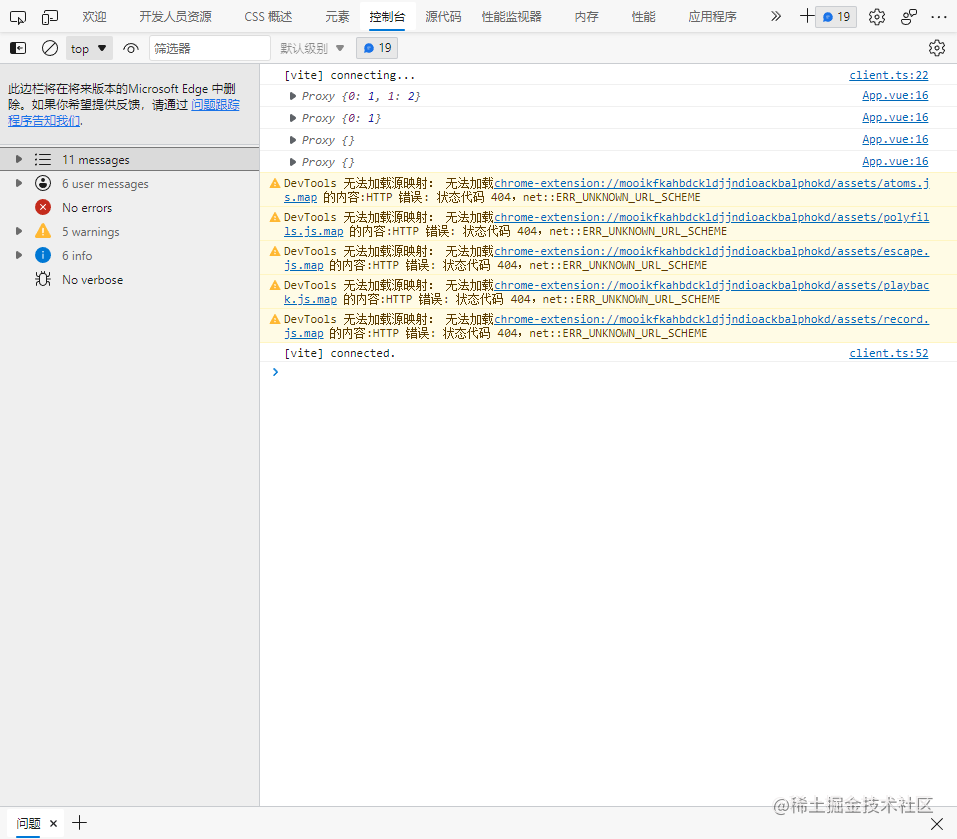``````    const array = ref([1,2,3]);

watch(array,()=>{
console.log(array.value);
},{
deep:true
})

array.value.splice(0,array.value.length)
``````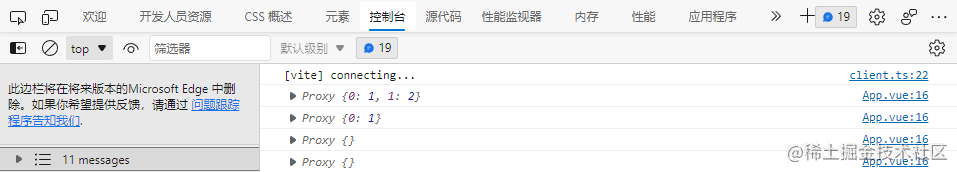# 3. 总结